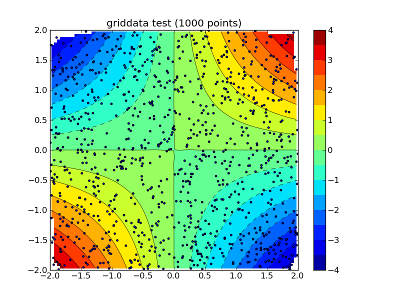# griddata question

Hi all,

what is the reason for the white areas in the corners of the interpolation domain ?
Any idea ?

Nils

import numpy as np
from scipy.interpolate import griddata
import matplotlib.pyplot as plt
import numpy.ma as ma
from numpy.random import uniform, seed
# make up some randomly distributed data
seed(1234)
npts = 1000
x = uniform(-2,2,npts)
y = uniform(-2,2,npts)
z = x*y
# define grid.
xi = np.linspace(-2.3,2.3,100)
yi = np.linspace(-2.3,2.3,100)
# grid the data.
zi = griddata((x, y), z, (xi[None,:], yi[:,None]), method='cubic')
# contour the gridded data, plotting dots at the randomly spaced data points.
CS = plt.contour(xi,yi,zi,15,linewidths=0.5,colors='k')
CS = plt.contourf(xi,yi,zi,15,cmap=plt.cm.jet)
plt.colorbar() # draw colorbar
# plot data points.
plt.scatter(x,y,marker='o',c='b',s=5)
plt.xlim(-2,2)
plt.ylim(-2,2)
plt.title('griddata test (%d points)' % npts)
plt.show()The white areas are not bounded by your data points (they are located
outside the convex hull of the data points), so the interpolation
algorithm would effectively be extrapolating.

Ryan

···

On Fri, Feb 18, 2011 at 1:24 PM, Nils Wagner <nwagner@...1052...> wrote:

Hi all,

what is the reason for the white areas in the corners of the interpolation
domain ?
Any idea ?

--
Ryan May